﻿ Does Temperature Effects the Growth of Microcracks in a Broken Femur with a Prosthetic Device AMBI?Publications are Open
Access in this journal
Article Versions
Export Article
• Normal Style
• MLA Style
• APA Style
• Chicago Style
Research Article
Open Access Peer-reviewed

### Does Temperature Effects the Growth of Microcracks in a Broken Femur with a Prosthetic Device AMBI?

Mary Tsili , D. Zacharopoulos
Biomedical Science and Engineering. 2017, 5(2), 14-18. DOI: 10.12691/bse-5-2-2
Published online: July 20, 2017

### Abstract

In present paper we considered if temperature effects the growth of microcracks in a broken femur with AMBI. We studied three particularly points of fracture. We used theory of adaptive elasticity neglecting and accounting temperature and energy density theory. We showed for both cases: that after a long time femur locally at points of our interest: i) will be (quickly or normally or delayed) united. Our results are verified by clinical studies. We concluded that temperature plays no role to growth of microcracks.

### 1. Introduction

The purpose of this paper is to study if temperature effects the growth of microcracks in a broken femur with AMBI. For this reason we will use the theory of adaptive elasticity 1, 2 neglecting and accounting temperature and energy density theory 3, 4, 5.

Macroscopically the bone has a volume V and a surface S. The volume V microscopically consists of microvolumeswhich generally are not homogenous 4, 5. Expanding theory of adaptive elasticity [ 1, p. 322] at microscopic area we assume:

i) A microvolume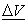of bone consists of an elastic microvolume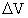(micromatrix bone) and of microporous (microcracks)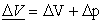(1)

where Δp is the volume of microcracks.

From the other hand Sih [ 4, p.179] showed that every microvolumecontains an homogenous microvolume. Thus we suppose that an elastic microvolume ΔV given by (1) is homogenous microvolume.

ii) The mechanical properties of microvolume of bone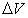coincides with the mechanical properties of homogenous microvolumeof micromatrix bone.

iii) The fraction of microvolume of the micromatrix boneis defined as [ 2, p. 322]: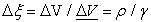(2)

where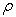is the density of microvolume, whileis the density of material (bone) and assume to be constant. From the above it follows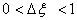.

v) The porosity that is the mean length of microcraks of microvolumealters with mass added /removal to /from micro matrix bone and linearly depends from the history of microstrain [ 1, p. 322]. The above is characterized by a parameter ê 2:(3)

where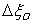is the initial fraction of the microvolume of micromatrix bone. With other words parameter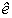is the change of the mean value of microcracks.

### 2. The Problem and Its Physical Approximation

Assume that someone breaks his /her left femur due to fall or traffic accident. Suppose that we deal with an intertrochanteric fracture type Α1 and case 3 [ 6, p.120; 7, p. 60]. The fracture starts from proximal femur and results to the last third of its diaphysis. We impose into injury bone a prosthetic device AMBI as indicates in Figure 1 and suggest to patient to stay at bed immobilization three months. We want to predict the situation of femur when patient start again walking, locally at three points of fracture area. The lasts have no contact with AMBI as indicated in Figure 2 and are the followings:

• Figure 1. Prosthetic device AMBI in a left broken femur
• Figure 2. A cylinder length L, with inner and outer radii a and b respectively and with AMBI device. The points Γ, Δ and Ε belong to fracture area (defined with intense black) but have no contact with AMBI

Γ: at middle distance between endosteal and periosteal surface and at the end of diaphysis of femur. The origin and end of the diaphysis of femur are defined as: nearest to joint knee and to proximal femur respectively.

Δ: at endosteal surface of femur and at 5/6 of the distance between origin and end of femur’s diaphysis.

Ε: at periosteal surface of femur and at 2/3 of the distance between origin and end of femur’s diaphysis.

At t=0 the last third of the diaphysis of femur was separated into two pieces due to fracture. Consequently all points that belong to fracture area ABEZ had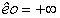and the same goes for points of our interest Γ, Δ and Ε. At t >0 we impose a prosthetic device AMBI into broken femur as indicated in Figure 1 and Figure2. We want to predict ê(t) after long time period at points Γ, Δ and Ε.

### 3. The Mathematic Modeling and the Solution of Our Problem

Femur’s diaphysis is modeled as a hollow circular cylinder with length L, inner and outer radii a and b respectively. These radii corresponds to endosteal and periosteal surfaces of bone and assume to be constant due to internal remodeling 8. Since we deal with microscopic area, we use the density energy theory 3, 4, 5.

The equations of the above theory in cylindrical coordinates are:

i) the stress relations between macroscopic and microscopicarea [ 4, p. 182]where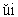,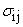and (dV/dA) are respectively: the macroscopic displacement, the stress on microvolume, the stress at macrovolume and the change of volume with surface 4, 5

ii) the macrostress - equations [ 5, p.182]:iii) the microstress equations [ 5, p.182]:iv) the microstrain-microdisplacement relations [ 5, p.179):v) the microstress - microstrain relations:where: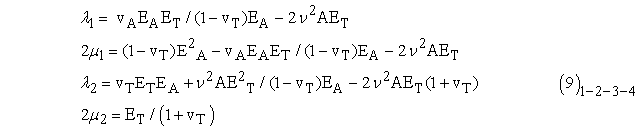where ΕΑ, ΕΤ and vA, vΤ are Young’s modulus and Poisson ratio in transverse and axial direction at macroscopic area.

Finally rate remodeling equation 2 at microscopic area without /and accounting temperature are respectively:where AT(ê), ΑΑ(ê) are rate remodeling coefficients in transverse and axial direction respectively while B(ê) is a rate remodeling coefficient depends from temperature.

The boundary conditions of our problem are:

i) at point Γ:ii) at point Δ: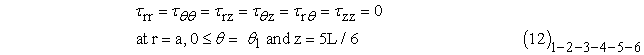iii) at point Z:Our problem has a unique solution [ 5, p.186] and assume that microdisplacements are of the form:where A(t), B(t), C(t) are unknowns. Then (7) are written as:Therefore microstress - microstrains relations (8) because of (12) take the forms: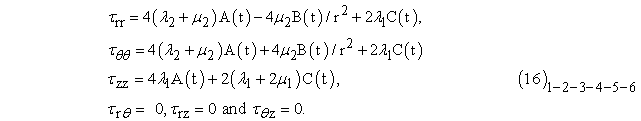Applying the boundary conditions (11)-(12)-(13) into (16) it is possible to obtain unknown functions A(t), B(t) and C(t):

i) at point Γ: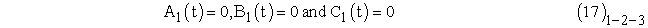ii) at point Δ: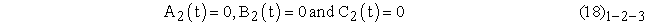iii) at point E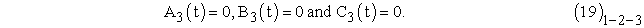Employing (17)-(18)-(19) into (14) it is possible to calculate microdisplacements for points Γ, Δ and Ε. Also (15) due to (17)-(18)- (19) become:

i) at point Γ:ii) at point Δ:iii) at point Ε: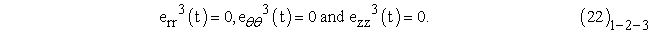At continuity we distinguish the following cases:

i) Internal remodeling of femur does not depends upon temperature:

Then substituting (20), (21), (22) into (10)1 it follows:

i) at point Γ: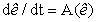(23)

ii) at point Δ:(24)

iii) at point Ε:(25)

At continuity we impose 9, 10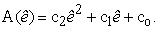(26)

Therefore (23)-(24)-(25) conclude to the following form: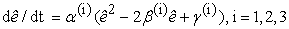(27)

where:

i) at point Γ:ii) at point Δ:iii) at point Ε: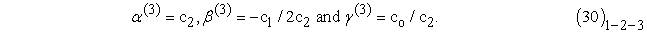The solution of (27) must satisfy the following condition(31)

since the mean length of microcracks can not be negative.

From the other hand (27) satisfies the initial condition: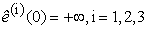(32)

because as we stated earlier points Γ, Δ and Ε belong to fracture area. We definedand distinguish the following cases 10:

1)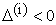. The solution of (27) that satisfies (32) is: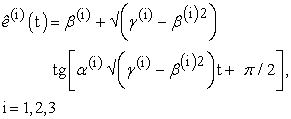(33)

i) If, then for, then it results that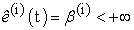. We distinguish the following subcases: ia) If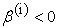the solution has no physical sense, since contradicts to (31). ib) If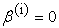then when the patient get of the bed immobilization, femur at points Γ, Δ and Ε will be under osteopetrosis. Ic) Finally if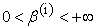, then when patient get of bed immobilization, femur at points Γ, Δ, Ε will be united. In addition if value of parameter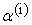is great, then value of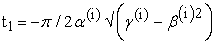is small. The last means that femur at points of our interest will be quickly united. If value of parameter α(i) is small, then the value ofis great. The last means that femur at points of our interest will be normally united.

ii) If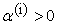, then for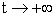it follows that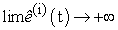. The last means that femur at points Γ, Δ and Ε, may be under a delayed union (healing).

iii) If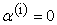then. This is the worst case because it means that the femur at points of our interest has not a union (healing).

2)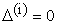. The solution of (27) is: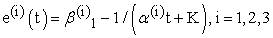(34)

i) If K = 0, then employing initial condition (32) into (34) it results that. The last contradicts to (32) and there is no solution. ii) If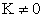, then employing initial condition (32) into (34) it possible to obtain. Specially if K>0 then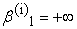, that isand we coincide with case 1. iii). From the other hand if K<0 then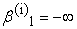, that is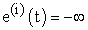and we coincide with case i).

3)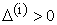. Then the solution of (27) that satisfies (32) is: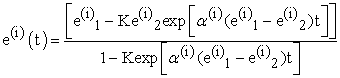(35)

where:From the above it follows that: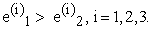(37)

Employing the initial condition (32) into (37) it follows: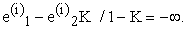(38)

From the above we conclude that K=1 and. The last contradicts to (37). Therefore (35) has no solution.

The solutions for finite and infinite t are in Table 1. and Table 2. respectively. Accordingly to all that results when patient get of the bed immobilization, his (hers) femur at points of our interest will be: quickly, or normally, or delay united, or it will under osteopetrosis, or will be not united.

ii) Internal remodeling depends upon temperature:

Τhen (10)2 because of (20), (21), (22) for all points Γ, Δ and Ε is written as:(39)

Finally substituting (26) and(40)

into (39) we again result to (27), where:

i) at point Γ:ii) at point Δ:iii) at point Ε:The solution of (27) satisfying initial condition (32) are given by (33), (34) and (35). The acceptable solutions are the same as at previous case and are given by Table 1 and Table 2.

### 4. Discussion and Conclusion

Our theoretical results for both cases: neglecting and accounting temperature are verified by clinical studies. Papasimos [ 6, p. 92] and 11 studied 40 cases of broken femur with AMBI. All fractures were united (100%) in a period ranged from 45 days until six months. Particularly 24 fractures were united in 45 days (quickly union 60%) 13 of them in 3 months (normally union 32%) and rests in 6 months (delay union7.5%). The mean time of union for all cases was 3.15 months and corresponds to normal union [ 11, p.93].

Also other examples of quick union to humans diaphysis and to animals methaphysis has been reffered 12, 13, 14. The acceptable solutions are in Table 3. From the above we result that temperature plays no role to growth of microcracks in a broken femur with prosthetic device AMBI.

### References

  Cowin S. and Hegedus D. (1976). “Bone remodeling I: Theory of adaptive elasticity”. J. Elastic. 6, pp. 313-326. In article View Article  Hegedus D. and Cowin S. (1976). Bone remodeling II: Theory of adaptive elasticity” J. Elastic. 6, pp. 337-352. In article View Article  Sih G. (1972-1982). “Mechanics of fracture, Introductory Chapters”, Vol. I- VII, edited by G.C. Sih, Martinus Nijhoff, The Hague. In article  Sih G.. (1985). “Mechanics and Physics of energy density theory”, Theoret., Appl., Fract., Mech., 44, pp. 157-173. In article View Article  Sih GC (1988). “Thermomechanics of solids: nonequilibrium and irreversibility”, Theoretical and Applied Fracture Mechanics, 9, pp. 175-198. In article View Article  Papasimos S. (2005). Phd Thesis. University of Patras, Greece. Also in: KATAΓΜΑ/69.pdf (in Greek). In article  Muller ME., Nazarian S., Koch P, Schatzker J. (eds) (1990). “The comprehensive classification of fractures of long bones.” Springer, Berlin, Heidelberg, New York, p 120. In article  Frost H.M (1964). “Dynamics of bone remodeling in bone biodynamics” (edited by Frost H.M) Little and Brown 316, Boston‘. In article PubMed  Cowin S. and Van -Burskirk W. (1978). “Internal bone remodeling induced by a medullary pin”. J. Biomech. 11, pp. 269-275. In article View Article  Tsili M. (2000). “Theoretical solutions for internal bone remodeling of diaphyseal shafts using adaptive elasticity theory” J. Biomech. 33 pp. 235-239. In article View Article  Papasimos S., Koutsojannis M., Panagopoulos A., P. Megas P. and Lambiris E. (2005). “A randomised comparison of AMBI, TGN and PFN for treatment of unstable trochanteric fractures, Arch Orthop. Trauma 125: 462-468. In article View Article  PubMed  in http/: Downloads/110.pdf:Giannakopoulos C. “Subtract of Osteosynthesis Materials. Indications and their Risk. Laboratory of Rechearch of Injuries Myoskeletal System, Athens University pp. 1-89. In article  in http/: “Fractures and their Treatment.pdf. Malizos K. “Orthopaedics Clinic, University o f Thessaly”. Also in: www.ortho.uth.org. In article  in http/: Porosi.pdf. Patsikas M.. “Ray’s Estimation of union of the fractures, Animals Medicine School, University of Thessaloniki, pp. 1-89. In articleThis work is licensed under a Creative Commons Attribution 4.0 International License. To view a copy of this license, visit http://creativecommons.org/licenses/by/4.0/